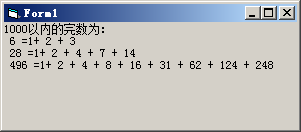# VB程序题：如果一个整数的所有因子（包括1，但不包括本身）之和与该数相等，则称这个数为完数。例如6＝1+2+3，所以6是一个完数。编写一个函数IsWs(m)判断m是否为完数，函数的返回值是逻辑型。……

VB程序题：如果一个整数的所有因子（包括1，但不包括本身）之和与该数相等，则称这个数为完数。例如6＝1+2+3，所以6是一个完数。编写一个函数IsWs(m)判断m是否为完数，函数的返回值是逻辑型。主调程序在列表框显示1000以内的完数，如图F.4所示。

Dim a%()
Private Sub Form_Click()
Print "1000以内的完数为："
For i = 1 To 1000
If IsWs(i) Then
Print i; "=1";
For j = 1 To UBound(a)
Print "+"; a(j);
Next j
Print
End If
Next i
End Sub
Function IsWs(m) As Boolean
Dim s%
s = 0
For i = 1 To m \ 2
If m Mod i = 0 Then
ReDim Preserve a(j)
a(j) = i
j = j + 1
s = s + i
End If
Next i
If m = s Then IsWs = True
End FunctionVisual Basic程序设计教程(第3版) (龚沛曾等编)课后实验源码

<< 上一篇 下一篇 >>